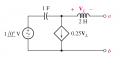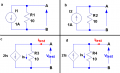# Finding Thévenin/Norton Impedence in a Circuit with Dependent Source

Thread Starter

#### ElectronicGuru

Joined Sep 26, 2014
22
Here's the circuit for which I need to find the Norton equivalent:I learned that in the case where we have a dependent source in the network to be simplified, we need to find Voc and Isc to find Zth = Voc/Isc (or Rth if this wasn't a phasor analysis problem). My question is: Why can't we find Zth by making the network inactive and finding the equivalent Zth of the inactive network?

In the above example, if the independent source is replaced by a short circuit, then doesn't this mean that the network is completely dead, i.e., VL must be zero? If so, then the voltage-controlled current source is really an open circuit, so Zth is simply the series combination of j2 - j = j Ω (given that ω = 1 rad/s). I know that this can't be right because using Voc/Isc (after completing phasor analysis), Zth = 0.5 + j Ω.

I'm looking for a general, intuitive reason. Thanks in advance for any help!

Last edited:

#### dalam

Joined Aug 9, 2014
58
In this sort of circuits I prefer to use a test source applied across the branch AB. Short circuit all the voltage source and open all the current source. This way the circuit does not become dead and the dependent source are analysed with ease.Thread Starter

#### ElectronicGuru

Joined Sep 26, 2014
22
In this sort of circuits I prefer to use a test source applied across the branch AB. Short circuit all the voltage source and open all the current source. This way the circuit does not become dead and the dependent source are analysed with ease.I do know about that technique, but I was hoping for an explanation why my method doesn't work for circuits with dependent sources. Probably something really obvious.

#### WBahn

Joined Mar 31, 2012
26,398
I do know about that technique, but I was hoping for an explanation why my method doesn't work for circuits with dependent sources. Probably something really obvious.
First, you need to ask why the method of turning off all the sources and calculating the impedance looking into terminals works when there are only independent sources. The short answer is that the rules you use to find the impedance are based on the relationships between voltage and current in passive elements and, although it doesn't seem like it, you really are applying a test voltage and determining the test current and taking the ratio of the two -- it's just that you've learned how to do this in a shortcut way for the various possible combinations of these components.

You can turn off all of the independent sources because the circuit is linear and so the effect of the independent sources is independent from the effect of the test source you are using (even when you don't realize you are using it). But the effect of a dependent source is dependent upon all of the other sources in the circuit. Since it is linear, you can deal with them one at a time but the key point is that it depends upon the test source used to find the Thevenin impedance and therefore has to be taken into account when this analysis is done. The most reasonable way to do this is to explicitly apply a test voltage and analyze the circuit to find the test current and then take the ratio of the two.

#### Jony130

Joined Feb 17, 2009
5,221
View attachment 76494
Why can't we find Zth by making the network inactive and finding the equivalent Zth of the inactive network?
Because you have a dependent source in your circuit. But the real answer is:
Circuit with dependent sources do not have Rth =Vth/Isc = constant.
See this examples:For circuit a and b we simply have
Rth = Vth/Isc = 10V/1A = 10Ω and Rth = Vth/Isc = -10V/-1A = 10Ω

But for circuit c and d situation looks different.
Vtest = 1V
Itest = 2*0.1A + 0.1A = 0.3A

So
Rth = Is*R3/(Is + 2Is) = Is*R3/(3Is) = R3/3 = 1V/0.3A = 3.33Ω

Now Rth for circuit d

Vtest = Is*R4
Itest = 0.1A - 0.2A = -0.1A
Rth = Is*R4/(Is - 2Is) = Is*R4/(-1Is) = R4/-1 = 1V/-0.1A= - 10Ω

As you can see Rth is not constant for a circuit with dependent sources. And this is why we cannot turn-off the dependent sources.

#### WBahn

Joined Mar 31, 2012
26,398
As an aside, the coefficient on the dependent current source in your diagram needs to have units of conductance, namely amps/volt. As written, it claims that the output current is equal to a voltage, which is nonsensical. A similar observation applies to the current-controlled voltage source in the diagrams posted by dalam. These should have units of impedance since the output is a voltage in response to a current in. As stated, it is saying that the output voltage is equal to a current, which is also nonsensical.

In addition to just being plain wrong, not giving the units makes it ambiguous. Is it amps/volt or perhaps is milliamp/volt or amps/millivolt or something else.

Also, if you patch up the author's sloppiness (and remember, most authors have never worked outside academia and so they just do not fully comprehend that a wrong answer in the real world has real world consequences, including death, destruction, and criminal and civil lawsuits for same), the you can track the units properly throughout your work (something that everyone one should always do, but unfortunately almost everyone was taught by people who, like the textbook authors, have never worked outside academia) to catch the vast majority of the mistakes you WILL make on an all too frequent basis (as we all do).

#### Jony130

Joined Feb 17, 2009
5,221
Sorry for confusion WBahn, and as a side note. In my diagram in examples C and D I use current controlled current source. So the coefficient has a dimension of A/A (current amplifier). CCCS output current is two times larger than R3 resistor current.

#### WBahn

Joined Mar 31, 2012
26,398
Sorry for confusion WBahn, and as a side note. In my diagram in examples C and D I use current controlled current source. So the coefficient has a dimension of A/A (current amplifier). CCCS output current is two times larger than R3 resistor current.
Notice that I didn't say anything about your diagrams, because the coefficients are correct (even if, in a textbook especially, it would almost certainly have been by sheer coincidence). While I might prefer to be explicit and use A/A or mA/mA, it is not incorrect to leave them off and I often do, particularly for voltage gains. You also tracked your units through your work, for which I applaud you.

Similar threads